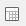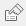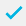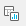# Creating a frequency table

Frequency tables show the response frequency for each available answer to a question. They are an easy method of analysing single questions in tabular form. The analysis table produces results by specifying the name of the question or questions. The data can be calculated as counts or percentages. The results can be filtered to look at subsets of data.

## Creating a frequency table from a single variable

The example below uses ‘Q4: Which of the following items did you order?’ in the Crocodile Rock Cafe survey supplied with Snap XMP Desktop.

1. Clickto display the Analysis Definition dialog box. Type Q4 in the Analysis field.
2. Click OK to create a frequency table that shows counts only.
1. Check Base Percents to express all answers as a percentage of the total number of respondents.
2. Select Analysis Base in the Order By drop-down list, so that the most frequently answered reply will be at the top of the table.
3. Click the Cells tab and check Suppress zeroes to hide any answers where the response count has been zero. (This option is normally used when a long list of codes exists for a question).
1. Click OK. This builds the frequency table.
1. Notice that the calculated percentages add up to a number greater than 100 because this question has a Multiple Response, so there are more responses than there are respondents.
2. To calculate the percentages to add up to 100, clickand select the Base/Labels tab.
3. Change the Base field from Respondents to Responses. Rebuild the table to alter the method of calculation.
1. Clickto save the table. The name will be generated by Snap. If you then wish to work on the table again, it is stored in the Analyses Window.

## Creating a frequency table showing two variables

If you use more than one variable in a frequency table, then you can:

• display the details for the variables side by side (using the keyword WITH)
• look at each permutation of the two variables using the key word PER

The example uses the frequency of visit question (Q2) and the gender question (Q12) in the same table.

## Displaying the variables next to one another

1. Clickto display the Analysis Definition dialog.
2. Type Q2:Q12 into the Analysis field. Note that : is the short form of the key word WITH, which means that Q2 is analysed with Q12 next to it.
3. Check Base Percents to express all answers as a percentage of the Base figure.
1. Check Transpose to align the table horizontally rather than vertically.
2. Click OK to build the table.

## Looking at each permutation of the variables

1. Click onto alter the specification of the table.
2. Change the text in the analysis field to Q2 PER Q12.
3. Click OK to build the amended table.

Note that the table shows all the male variations of the frequency of visit, followed by all the female variation of the frequency of visit.

1. Clickto save the table. The name is generated automatically. The table can be edited using the Analyses Window.
Contents